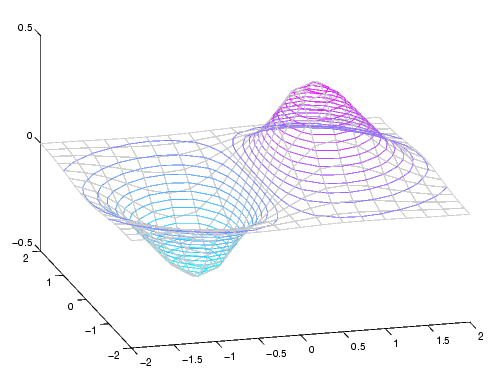MATLAB Function Referencecontour3

Three-dimensional contour plot

Syntax

• ```contour3(Z)
contour3(Z,n)
contour3(Z,v)
contour3(X,Y,Z)
contour3(X,Y,Z,n)
contour3(X,Y,Z,v)
contour3(axes_handle,...)
contour3(...,`LineSpec`)
[C,h] = contour3(...)
```

Description

`contour3` creates a three-dimensional contour plot of a surface defined on a rectangular grid.

```contour3(Z) ``` draws a contour plot of matrix `Z` in a three-dimensional view. `Z` is interpreted as heights with respect to the x-y plane. `Z` must be at least a 2-by-2 matrix. The number of contour levels and the values of contour levels are chosen automatically. The ranges of the x- and y-axis are `[1:n]` and `[1:m]`, where `[m,n] = size(Z)`.

```contour3(Z,n) ``` draws a contour plot of matrix `Z` with `n` contour levels in a three-dimensional view.

```contour3(Z,v) ``` draws a contour plot of matrix `Z` with contour lines at the values specified in vector `v`. The number of contour levels is equal to `length(v)`. To draw a single contour of level `i`, use `contour(Z,[i i])`.

```contour3(X,Y,Z), contour3(X,Y,Z,n), and contour3(X,Y,Z,v) ``` use `X` and `Y` to define the x- and y-axis limits. If `X` is a matrix, `X(1,:)` defines the x-axis. If `Y` is a matrix, `Y(:,1)` defines the y-axis. When `X` and `Y` are matrices, they must be the same size as `Z`, in which case they specify a surface as `surf` does.

```contour3(...,LineSpec) ``` draws the contours using the line type and color specified by `LineSpec`.

```contour3(axes_handle,...) ``` plots into the axes with handle `axes_handle` instead of the current axes (`gca`).

```[C,h] = contour3(...) ``` returns the contour matrix `C` as described in the function `contourc` and a column vector containing handles to graphics objects. `contour3` creates patch graphics objects unless you specify `LineSpec`, in which case `contour3` creates line graphics objects.

Remarks

If you do not specify `LineSpec`, `colormap` and `caxis` control the color.

If `X` or `Y` is irregularly spaced, `contour3` calculates contours using a regularly spaced contour grid, then transforms the data to `X` or `Y`.

Examples

Plot the three-dimensional contour of a function and superimpose a surface plot to enhance visualization of the function.

• ```[X,Y] = meshgrid([-2:.25:2]);
Z = X.*exp(-X.^2-Y.^2);
contour3(X,Y,Z,30)
surface(X,Y,Z,'EdgeColor',[.8 .8 .8],'FaceColor','none')
grid off
view(-15,25)
colormap cool```

See Also

`contour`, `contourc`, `meshc`, `meshgrid`, `surfc`

Contour Plots category for related functions

Contour Plots section for more examples

© 1994-2005 The MathWorks, Inc.